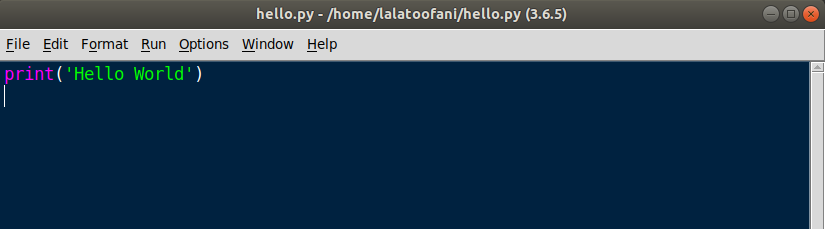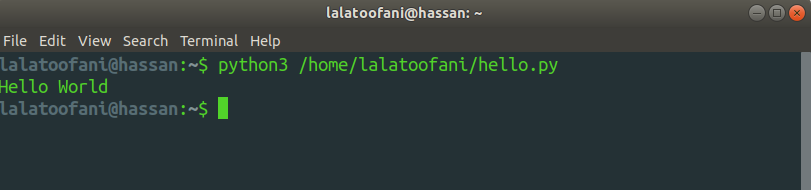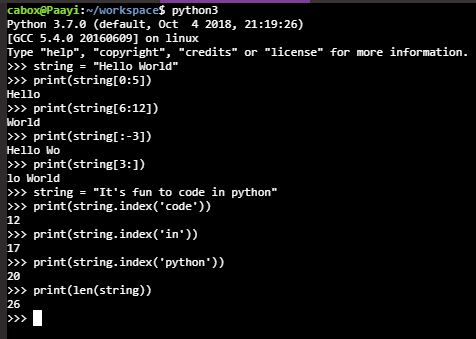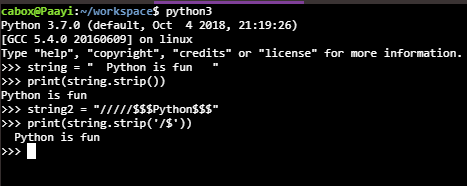## Python Tutorials: Write your First Program 'Hello World' With Python

##### Python Tutorials: Learn how to Code your First program in Python
Written by |15-Sep-2020 | 0 Comments | 3505 Views

Now the python is running in your systems; let's dive into python without wasting any more time.

Now let's Write Your First program of Printing' Hello World':

Open you're idle and go to the 'Files' tab and open a new file.

Save this file as 'hello.py.' and write the code as follows:

 print ('Hello World') Or string = "Hello World." print (string)And go to the 'Run' option on the taskbar and click on the run module, or you can directly run by pressing the F5 key.

The second option is running from the terminal. The following example is given in the Linux terminal, but it can be executed from all other operating systems.The same command will be run on Mac, but windows use "python" instead of "python3".

What are Variables in Python:

In python, there is a strict pattern to name the variables. Users cannot name a variable in any fashion. We can only use characters, numbers, and underscore.

The number should not be used at the starting of the variable. Following are some example to name the variables:

 variable1 = 'string' variable_1 = 'string' variable_var = 'string'

This is the right way to name a variable.

A syntax error will be generated if we named a variable in the wrong way as following:What are Strings in Python:

Unlike C, C++, and Java, there is no specific data type before initializing the variable. Python will decide on run time weather the variable is String, Integer, or Float.

So, at first, we will have a look at how we can initialize strings.

How to do String Initialization:

In python, the string can be initialized by the following methods:

 #Method 1: string1 = "This is the string in double-quotes." #Method 2: string2 = 'This the second string in single quotes.' #Method 3: string3 = "If you want to use single quotes then use like 'this'" #Method 4: string4 = '''You can also write multiple lines in python. To write such lines, you have to use triple quotes.'''

How to do String Slicing:

We can slice the strings in python; this procedure is known as string slicing in programming. String slicing accepts the starting index and ending index within square brackets.

Note: The index starts from 0

Following are the examples of slicing the strings:

 string = "Hello World" print(string[0:5]) #Hello print(string[6:12]) #World print(string[:-3]) #Hello Wo print(string[3:]) #lo WorldWe can also get the index of a specific word in a string by running the following program:

 string = "It's fun to code in python" print(string.index('code')) #12 print(string.index('in')) #17 print(string.index('python')) #20

The following are the indexes at which these words are starting from. We can also get the length of the string by python built-in function len(). It is to be noted that the length function starts from 1, whereas the index starts from 0.

 string = "It's fun to code in python." print(len(string)) #26

What is String Manipulation?:

String manipulation is a technique to change the string according to the need. We can remove the characters from the string, split the string into half, and search for the characters in a string.

How to String.split():

 string = "It's fun to code in python" print(string.split(' ')) #["It's", 'fun', 'to', 'code', 'in', 'python']   string2 = "It's/fun/to/code/in/python" print(string2.split('/')) #["It's", 'fun', 'to', 'code', 'in', 'python']

Split functions take one argument and split the string on that argument. If that argument is present in the string, then that string will be splinted; otherwise, nothing will happen. The split function returns the value as a list. We will see in the latter part that what is list and how to handle the list.String.strip():

Strip function takes the argument and removes that value from that string. By default, if no parameter is passed in the strip function, then it removes the starting and ending spaces of string; otherwise, it removes that particular character from that string.

Following is the example of stripping function:

 string = "  Python is fun   " print(string.strip()) #Python is fun   string2 = "/////\$\$\$Python\$\$\$" print(string.strip('/\$')) #Python is funString.replace():

The replace function replaces the value from a particular string. It takes two arguments. The first one is the character that is to be replaced and the other character that will be the value of that replacement.

 string = "Python programming is fun." print(string.replace('fun','cool')) #Python programming is cool

Upper and Lower:

The upper and lower function converts the string from lower case to upper case and from upper case to lower case in the following scenario. These are used for string manipulation.

 string = "Python programming is fun." print(string.upper()) # PYTHON PROGRAMMING IS FUN   string2 = "PYTHON PROGRAMMING IS FUN" print(string.lower()) # python programming is fun

Why are these string manipulations used?

This string manipulation is used to clean the data. In advance topics, when we will be studying data science, this string manipulation will be extensively used to clean the data and organize the data in a format in which we want to see it. Moreover, these are used to see special characters whether they are present in a string or not or to make a long sentence into words, as we see in the example of a splitting function.

Concatenation:

Concatenation is a process to add two strings to form as one string. In python, there are two different ways to concatenate the strings. One way of concatenating the string is to use the plus (+) operator between two strings, and the other way of doing so is to use a comma(,) symbol between two strings. The following method is only applicable when printing the two variables.

 a = "Hello" b = "World" res = a+b res_space = a+' '+b print(res) #HelloWorld   print(res_space) #Hello World   Concatenation with coma: print(a,b) #Hello World

String Formatting:

String formatting is a technique to create a new string by adding the variable without concatenating. There are several ways of formatting the string in python. Following is the way to implement the formatting of the string.

The first example is called c-style formatting. In this formatting, we define the string value by "%s" and for integer "%d" is used. The way to implement the method is as follows:

 print("Hello %s, today's weather is %d"%('folks',43)) #Hello folks, today's weather is 43

The second example of doing so is by using a format function. In this method, curly braces are made within a string, which is replaced by the variable when it is printed.

 print("Hello {}, today's weather is {}".format('folks',43)) #Hello folks, today's weather is 43

The third technique is more accessible than the examples mentioned above, and we use "f" at the start of the string two specify the formatting, which is as follows:

 name = "folks" temp = 43 print(f"Hello {name}, today's weather is {temp}") #Hello folks, today's weather is 43